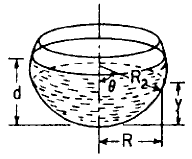Related Resources: pressure-vessel

### Spherical Cylinder Stress and Deflection Equations and Calculator

Spherical Cylinder Stress and Deflection Filled to depth d with liquid of density d force/unit volume; tangential edge support Equation and Calculator.

Per. Roarks Formulas for Stress and Strain for membrane stresses and deformations in thin-walled pressure vessels.

 Spherical Cylinder Stress and DeflectionFIlled to Depth ​With LiquidDeflection and Stress Filled to depth d with liquid of density d force/unit volume; tangential edge support .

For R2 / t > 10

At any level y below the liquid surface, y < d

Meridional StressCircumferential Hoop StressChange in height dimension yRotation of a meridian from its unloaded positionWeight of liquidWhere used:
E = Modulus of Elasticity (lbs/in2)
v = Poisson's ratio
δ = Density (lbs/in3)
σ1,2 = Stress, (lbs/in2)